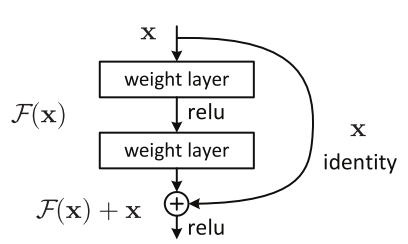# Resnet implementationResent

``````class ResNetBlock(nn.Module):
"""docstring for ResNetBlock"nn.Module"""
def __init__(self, input_channels):
super(ResNetBlock,self).__init__()

self.bn = nn.BatchNorm2d(input_channels, eps=0.001)

def forward(self, x):
x_previous = copy.deepcopy(x)
x = self.branch_1_3x3(x)
x = self.bn(x)
x = self.branch_1_5x5(x)
x = self.bn(x)
return F.relu(output, inplace=True)
``````

I’m getting this error

Traceback (most recent call last):
File “irbc1.py”, line 342, in
model_ft = train_model(net,criterion,optimizer_ft,exp_lr_scheduler,num_epochs=10)
File “irbc1.py”, line 295, in train_model
outputs = model(inputs)
File “/home/ffffff/.virtualenvs/LearnPytorch/lib/python3.6/site-packages/torch/nn/modules/module.py”, line 477, in call
result = self.forward(*input, **kwargs)
File “irbc1.py”, line 123, in forward
x = self.ResNet_1(x)
File “/home/ffffff/.virtualenvs/LearnPytorch/lib/python3.6/site-packages/torch/nn/modules/module.py”, line 477, in call
result = self.forward(*input, **kwargs)
File “irbc1.py”, line 143, in forward
x_previous = copy.deepcopy(x)
File “/home/ffffff/.virtualenvs/LearnPytorch/lib/python3.6/copy.py”, line 161, in deepcopy
y = copier(memo)
File “/home/ffffff/.virtualenvs/LearnPytorch/lib/python3.6/site-packages/torch/tensor.py”, line 15, in deepcopy
raise RuntimeError("Only Tensors created explicitly by the user "
RuntimeError: Only Tensors created explicitly by the user (graph leaves) support the deepcopy protocol at the moment

What is going wrong here?

You don’t need to deepcopy here. Just assign `x` to another variable and add it later.
Have a look at the torchvision implementation.

Also you are reusing the `nn.BatchNorm` layer, which is most likely not the best idea.

``````    def forward(self, x):
residual = x

out = self.conv1(x)
out = self.bn1(out)
out = self.relu(out)

out = self.conv2(out)
out = self.bn2(out)

if self.downsample is not None:
residual = self.downsample(x)

out += residual
out = self.relu(out)
``````

can see batchNorm used here also two times
Thank you
then what is .clone() used for?

as we are programming in python, doesn’t the concept of shallow and deep copy apply here?

Sure, but two different `nn.BatchNorm` layers (`bn1` and `bn2`) instead of the same twice.

As you are not reassigning `x` to the output of the layers, it won’t be overwritten.

``````def conv_block(in_f, out_f, *args, **kwargs):
return nn.Sequential(
nn.Conv2d(in_f, out_f, *args, **kwargs),
nn.BatchNorm2d(out_f),
nn.ReLU()
)

class MyCNNClassifier(nn.Module):
def __init__(self, in_c, n_classes):
super().__init__()
self.conv_block1 = conv_block(in_c, 32, kernel_size=3, padding=1)

self.conv_block2 = conv_block(32, 64, kernel_size=3, padding=1)

self.decoder = nn.Sequential(
nn.Linear(32 * 28 * 28, 1024),
nn.Sigmoid(),
nn.Linear(1024, n_classes)
)

def forward(self, x):
x = self.conv_block1(x)
x = self.conv_block2(x)

x = x.view(x.size(0), -1) # flat

x = self.decoder(x)

return x
``````

Is this kind of BatchNorm fine?

Yes, looks good as both `conv_blocks` now have their own `BatchNorm` layer.